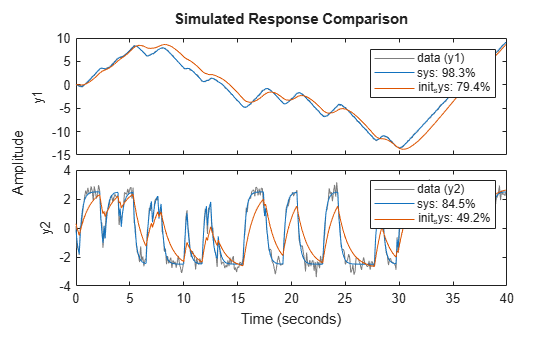# pem

Prediction error estimate for linear and nonlinear model

## Syntax

``sys = pem(data,init_sys)``
``sys = pem(data,init_sys,opt)``

## Description

example

````sys = pem(data,init_sys)` updates the parameters of an initial model to fit the estimation data. The function uses prediction-error minimization algorithm to update the parameters of the initial model. Use this command to refine the parameters of a previously estimated model.```

example

````sys = pem(data,init_sys,opt)` specifies estimation options using an option set.```

## Examples

collapse all

Estimate a discrete-time state-space model using the subspace method. Then, refine it by minimizing the prediction error.

Estimate a discrete-time state-space model using `n4sid`, which applies the subspace method.

```load iddata7 z7; z7a = z7(1:300); opt = n4sidOptions('Focus','simulation'); init_sys = n4sid(z7a,4,opt);```

`init_sys` provides a 73.85% fit to the estimation data.

`init_sys.Report.Fit.FitPercent`
```ans = 73.8490 ```

Use `pem` to improve the closeness of the fit.

`sys = pem(z7a,init_sys);`

Analyze the results.

`compare(z7a,sys,init_sys);``sys` provides a 74.54% fit to the estimation data.

Estimate the parameters of a nonlinear grey-box model to fit DC motor data.

Load the experimental data, and specify the signal attributes such as start time and units.

```load(fullfile(matlabroot,'toolbox','ident','iddemos','data','dcmotordata')); data = iddata(y, u, 0.1); data.Tstart = 0; data.TimeUnit = 's';```

Configure the nonlinear grey-box model (`idnlgrey`) model.

For this example, use `dcmotor_m.m` file. To view this file, type `edit dcmotor_m.m` at the MATLAB® command prompt.

```file_name = 'dcmotor_m'; order = [2 1 2]; parameters = [1;0.28]; initial_states = [0;0]; Ts = 0; init_sys = idnlgrey(file_name,order,parameters,initial_states,Ts); init_sys.TimeUnit = 's'; setinit(init_sys,'Fixed',{false false});```

`init_sys` is a nonlinear grey-box model with its structure described by `dcmotor_m.m`. The model has one input, two outputs and two states, as specified by `order`.

`setinit(init_sys,'Fixed',{false false})` specifies that the initial states of `init_sys` are free estimation parameters.

Estimate the model parameters and initial states.

`sys = pem(data,init_sys);`

`sys` is an `idnlgrey` model, which encapsulates the estimated parameters and their covariance.

Analyze the estimation result.

`compare(data,sys,init_sys);``sys` provides a 98.34% fit to the estimation data.

Create a process model structure and update its parameter values to minimize prediction error.

Initialize the coefficients of a process model.

```init_sys = idproc('P2UDZ'); init_sys.Kp = 10; init_sys.Tw = 0.4; init_sys.Zeta = 0.5; init_sys.Td = 0.1; init_sys.Tz = 0.01;```

The `Kp`, `Tw`, `Zeta`, `Td`, and `Tz` coefficients of `init_sys` are configured with their initial guesses.

Use `init_sys` to configure the estimation of a prediction error minimizing model using measured data. Because `init_sys` is an `idproc` model, use `procestOptions` to create the option set.

```load iddata1 z1; opt = procestOptions('Display','on','SearchMethod','lm'); sys = pem(z1,init_sys,opt);```

Examine the model fit.

`sys.Report.Fit.FitPercent`
```ans = 70.6330 ```

`sys` provides a 70.63% fit to the measured data.

## Input Arguments

collapse all

Estimation data that contains measured input-output data, specified as an `iddata` or `idfrd` object. You can use frequency-domain data only when `init_sys` is a linear model.

The input-output dimensions of `data` and `init_sys` must match.

Identified model that configures the initial parameterization of `sys`, specified as a linear, or nonlinear model. You can obtain `init_sys` by performing an estimation using measured data or by direct construction.

`init_sys` must have finite parameter values. You can configure initial guesses, specify minimum/maximum bounds, and fix or free for estimating any parameter of `init_sys`:

• For linear models, use the `Structure` property. For more information, see Imposing Constraints on Model Parameter Values.

• For nonlinear grey-box models, use the `InitialStates` and `Parameters` properties. Parameter constraints cannot be specified for nonlinear ARX and Hammerstein-Wiener models.

Estimation options that configure the algorithm settings, handling of estimation focus, initial conditions, and data offsets, specified as an option set. The command used to create the option set depends on the initial model type:

## Output Arguments

collapse all

Identified model, returned as the same model type as `init_sys`. The model is obtained by estimating the free parameters of `init_sys` using the prediction error minimization algorithm.

## Algorithms

PEM uses numerical optimization to minimize the cost function, a weighted norm of the prediction error, defined as follows for scalar outputs:

`${V}_{N}\left(G,H\right)=\sum _{t=1}^{N}{e}^{2}\left(t\right)$`

where e(t) is the difference between the measured output and the predicted output of the model. For a linear model, the error is defined as:

`$e\left(t\right)={H}^{-1}\left(q\right)\left[y\left(t\right)-G\left(q\right)u\left(t\right)\right]$`

where e(t) is a vector and the cost function ${V}_{N}\left(G,H\right)$ is a scalar value. The subscript N indicates that the cost function is a function of the number of data samples and becomes more accurate for larger values of N. For multiple-output models, the previous equation is more complex. For more information, see chapter 7 in System Identification: Theory for the User, Second Edition, by Lennart Ljung, Prentice Hall PTR, 1999.

## Alternative Functionality

You can achieve the same results as `pem` by using dedicated estimation commands for the various model structures. For example, use `ssest(data,init_sys)` for estimating state-space models.